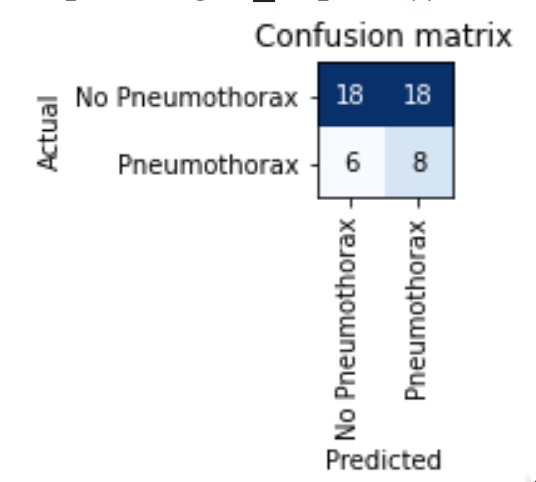# Beginner question on confusion matrix

I tried my own example with 20+ classes in the 02_production.ipynb. With this number of classes, the confusion matrix is not readable anymore. How can I increase the size of the confusion matrix? I tried the doc command, but there does not seem to be an option for the size (at least with fastai2 the figsize seems to be gone?).

Does anybody else have this problem? How did you solve it with fastai2?

Confusion matrix plot created using matplotlib. You would want to check out on how to tweak the figure sizes in matplotlib, and add the desired figure size before calling confusion matrix.

Hi ringoo hope all is well!

Maye the code below could help.

``````interp = ClassificationInterpretation.from_learner(learn)
losses,idxs = interp.top_losses()
len(dls.valid_ds)==len(losses)==len(idxs)
interp.plot_confusion_matrix(figsize=(1,1))
``````changing the above figsize(20,20)

produced this plot which is too big at 100% for my laptop browser.

This code is from fastai 2.
Hope this helps.
Cheers mrfabulous11 Like

You can increase the size of the confusion matrix plot but as Jeremy said when you have a big number of classes it will be hard to get a clear understanding using only the confusion matrix. Instead you should also use `interp.most_confused(min_val=n)`: it will show you the `n` most confused predictions and the actual labels in addition to the number the model has done this mistake.

1 Like

This really helped me, thank you!

1 Like